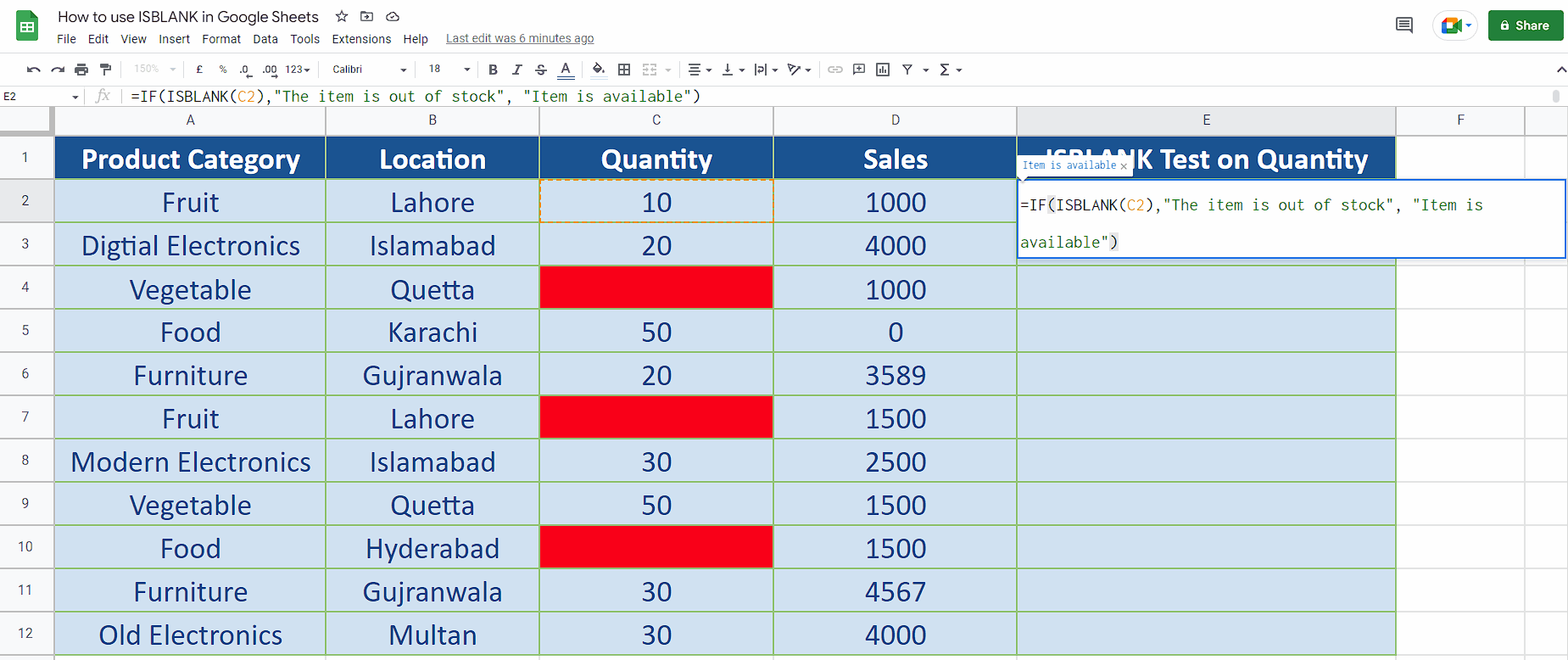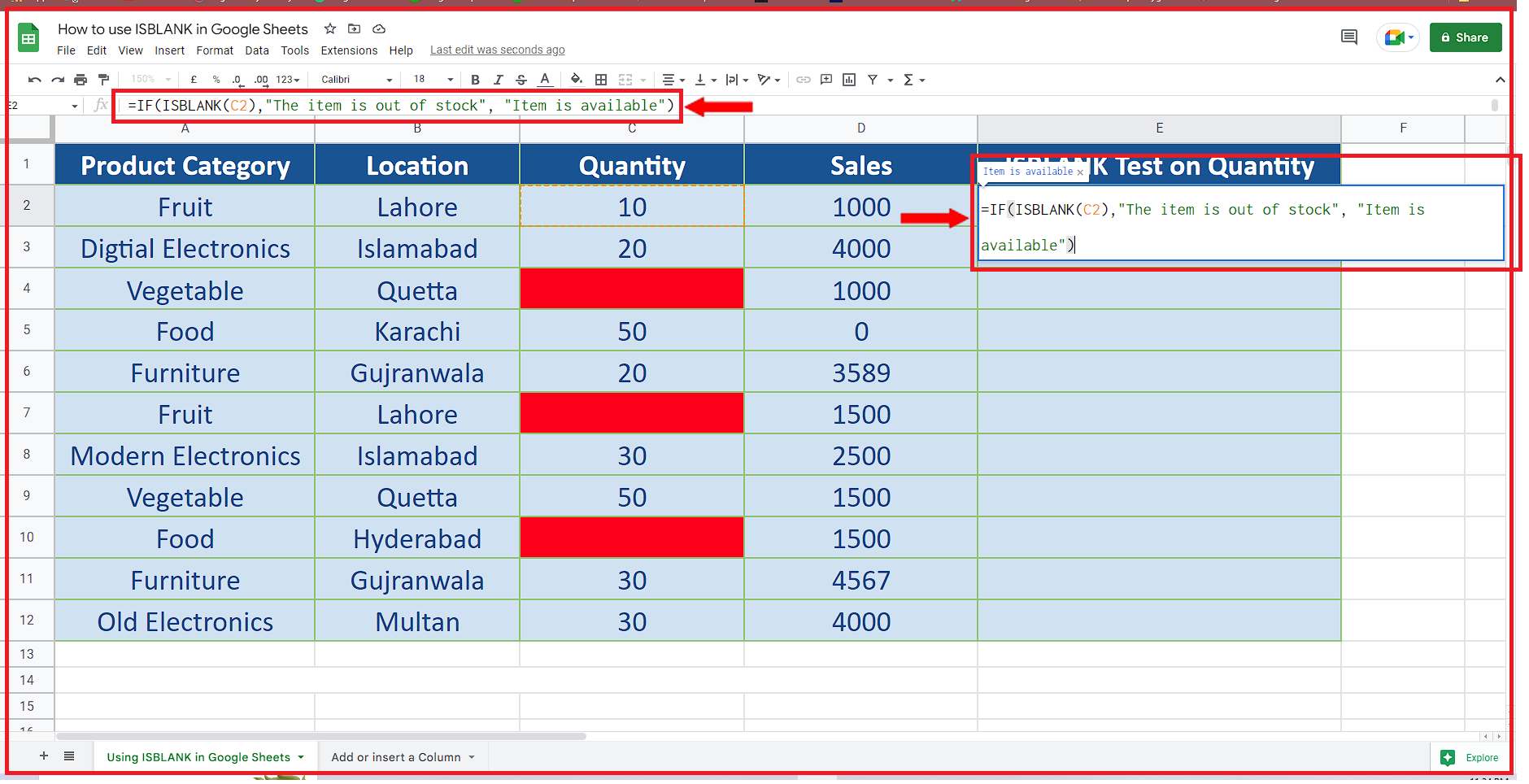# How to use ISBLANK in Google SheetsWhile working with Google Sheets or even Excel, sometimes we need to find out if a cell in the dataset is empty or blank because then we can take some actions based on this result. Therefore, Google Sheets and Excel both provide us with a function called ISBLANK. In today’s tutorial we’ll learn how to use the ISBLANK function in Google Sheets.

### Step 1 – Create the formula– We are going to use the ISBLANK function with IF statement to decide whether an item is available in the stock or not. So, we’ll use the following simple formula
=IF(ISBLANK(C2), “The item is out of stock”, “Item is available”)
This formula checks if the cell C2 is empty then The item is out of stock message otherwise Items is available message will be shown in the cell where we’ll write this formula as shown above.

### Step 2 – Implement the formula and extend to all data– After writing the formula press Enter key. This will implement the formula to the first cell.
– Now extend the formula by clicking and holding the fill handle and dragging it down to the last data row. This will implement the formula to all data values as shown above.